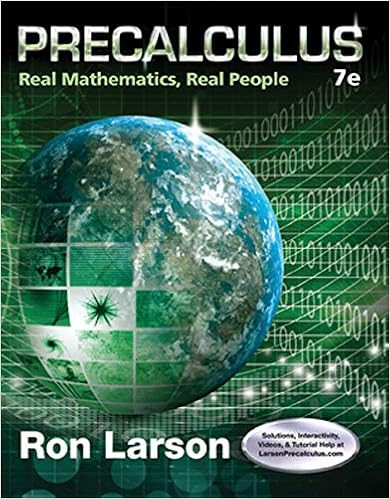# B the absolute values of 12 and 9 are 12 and 9 and 12

• Notes
• 84
• 100% (3) 3 out of 3 people found this document helpful

This preview shows page 27 - 30 out of 84 pages.

##### We have textbook solutions for you!
The document you are viewing contains questions related to this textbook.The document you are viewing contains questions related to this textbook.
Chapter 2 / Exercise 99
Precalculus: Real Mathematics, Real People
LarsonExpert Verified
b) The absolute values of 12 and 9 are 12 and 9, and 12 9 21. So, ( 12) ( 9) 21. c) Add the absolute values of 3.5 and 6.28, and put a negative sign on the sum. Remember to line up the decimal points when adding decimal numbers: 3.50 6.28 9.78 So ( 3.5) ( 6.28) 9.78. d) 1 2 1 4 2 4 1 4 3 4 Now do Exercises 1–10
##### We have textbook solutions for you!
The document you are viewing contains questions related to this textbook.The document you are viewing contains questions related to this textbook.
Chapter 2 / Exercise 99
Precalculus: Real Mathematics, Real People
LarsonExpert Verified
•••